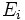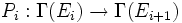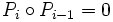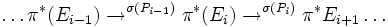# Elliptic complex

This article or section of article is sourced from:Wikipedia

## Definition

### Symbol-free definition

A differential complex is said to be elliptic if its sequence of symbols is exact.

### Definition with symbols

Let$M$ be a differential manifold and$E_i$ be smooth vector bundles over$M$. Let$P_i:\Gamma(E_i) \to \Gamma(E_{i+1})$ form a differential complex (viz$P_i \circ P_{i-1} = 0$. Then this differential complex is said to be elliptic if the following sequence of symbols is exact:$\ldots \pi^*(E_{i-1}) \to^{\sigma(P_{i-1})} \pi^*(E_i) \to^{\sigma(P_i)} \pi^*{E_{i+1}} \ldots$